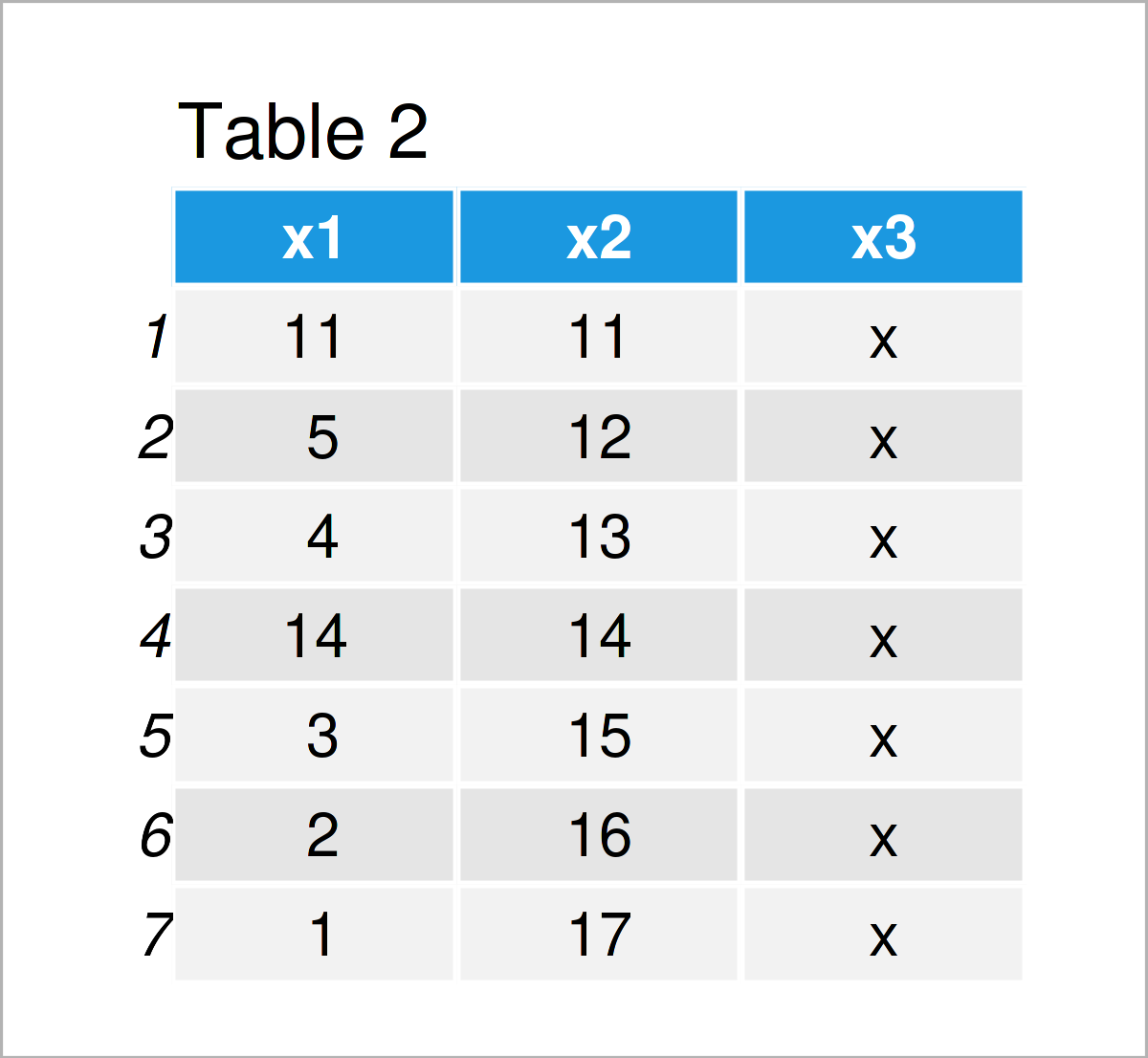# Replace NA Values in Column by Other Variable in R (Example)

In this post you’ll learn how to exchange NA values by another variable in the R programming language.

The tutorial contains these content blocks:

Let’s jump right to the tutorial.

## Creation of Example Data

At first, let’s create some example data:

```data <- data.frame(x1 = c(NA, 5, 4, NA, 3, 2, 1), # Create example data x2 = 11:17, x3 = "x") data # Print example data```Table 1 shows that our example data consists of seven rows and the three columns “x1”, “x2”, and “x3”. The variable x1 contains several missing values (i.e. NA values):

## Example: Replace NA Values in One Column by Another Column

This example illustrates how to exchange missing data in one column by the corresponding row values in another column of the same data frame.

To achieve this, we can use the is.na function as shown below:

```data_new <- data # Duplicate data data_new\$x1[is.na(data_new\$x1)] <- data_new\$x2[is.na(data_new\$x1)] # Replace NA values data_new # Print new data```By running the previous R programming code we have created Table 2, i.e. a new data frame where all NA values in x1 have been imputed by the values in x2.

## Video & Further Resources

Do you want to know more about the replacement of missings in a column by another variable? Then I recommend watching the following video on my YouTube channel. I’m explaining the R syntax of this post in the video:

In addition, you might want to read the related articles on this homepage.

To summarize: In this R tutorial you have learned how to substitute NA values in a column by another adjacent variable. Don’t hesitate to let me know in the comments, if you have additional questions. Besides that, please subscribe to my email newsletter for updates on the newest articles.

Subscribe to the Statistics Globe Newsletter